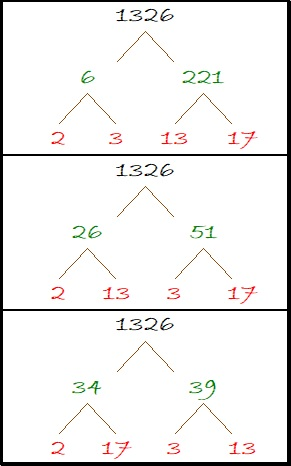# The Shape of 1326

1326 is the 51st triangular number because 26 × 51 = 1326 and 2(26) is 1 more than 51.

Since 51 is an odd number, 1326 is also a hexagonal number. It is the 26th hexagonal number because 26 is half of 52 which is 1 more than 51.

Triangular numbers aren’t hard to visualize, but hexagonal numbers might be. Here are 1326 tiny dots showing that 1326 is a hexagonal number:Here are some more facts about the number 1326:

• 1326 is a composite number.
• Prime factorization: 1326 = 2 × 3 × 13 × 17
• The exponents in the prime factorization are 1, 1, 1, and 1. Adding one to each and multiplying we get (1 + 1)(1 + 1)(1 + 1)(1 + 1) = 2 × 2 × 2 × 2 = 16. Therefore 1326 has exactly 16 factors.
• Factors of 1326: 1, 2, 3, 6, 13, 17, 26, 34, 39, 51, 78, 102, 221, 442, 663, 1326
• Factor pairs: 1326 = 1 × 1326, 2 × 663, 3 × 442, 6 × 221, 13 × 102, 17 × 78, 26 × 51, or 34 × 39
• 1326 has no square factors that allow its square root to be simplified. √1326 ≈ 36.41428Here are some factor trees for 1326 that won’t topple over.1326 is also the long side of four different Pythagorean triple triangles:
840-1026-1326 which is 6 times (140-171-221)
126-1320-1326 which is 6 times (21-220-221)
510-1224-1326 which is (5-12-13) times 102
624-1170-1326 which is (8-15-17) times 78

This site uses Akismet to reduce spam. Learn how your comment data is processed.